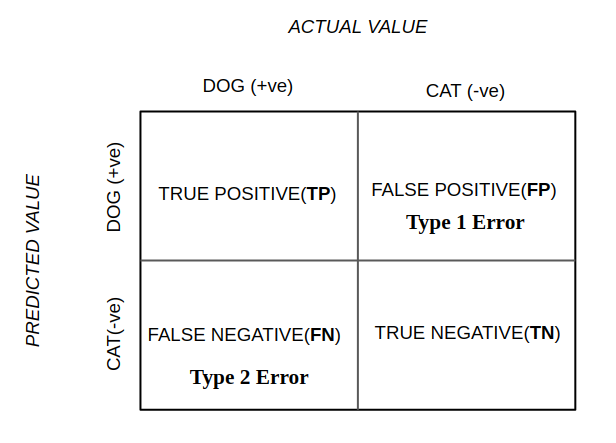# What is a confusion matrix?

What is a confusion matrix ?

We use the confusion matrix to evaluate the performance of a classification model. In this example, we are using a classifier to predict whether it is a dog or a cat. My main aim is to make you understand the intuition behind the terminologies.Confusion Matrix

TRUE POSITIVE (TP): Prediction is a dog and actually a dog.

TRUE NEGATIVE (TN): Prediction is a cat and actually a cat.

FALSE POSITIVE (FP): Prediction is a dog and actually a cat. Also known as “ TYPE I ERROR ”.

FALSE NEGATIVE (FN): Prediction is a cat and actually a dog. Also known as “ TYPE II ERROR ”.